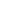# Free Â Class 10 Relationship between discriminants and nature of roots Worksheets

Download free printable Â Class 10 Relationship between discriminants and nature of roots Worksheets to practice. With thousands of questions available, you can generate as many Â Class 10 Relationship between discriminants and nature of roots Worksheets as you want.

## Sample Â Class 10 Relationship between discriminants and nature of roots Worksheet Questions

1.

Find the positive value of K for which equation will have equal roots.

2.

If equation has equal roots, prove that

3.

if x = -4 is a root of the equation , find the value of k so that the roots of the equation are equal.

4.

If x = -2 is a root of the equation , find the value of k so that the roots of the equation are equal.

5.

Find the value of k for which the equation has equal roots. Also find the roots.

6.

Find the value of P for which the quadratic equation  has equal roots.

7.

If the roots of the quadratic equation are equal prove that 2b = a + c

8.

Find the positive value of K for which equation and both will have equal roots.

9.

If roots of the equation are equal show that the roots of the equation are also equal.

10.

For what value of K will the equation have real and equal roots

Worksheets by UrbanPro

Our worksheets are designed to help students explore various topics, practice skills and enrich their subject knowledge, to improve their academic performance. Designed by Experts who have extensive experience and expertise in teaching a subject, these worksheets will improve your child's problem-solving skills and subject knowledge in a fun and interactive manner.
Check out our free customized worksheets across school boards, grades, subjects and levels of subject knowledge. You can download, print and share these worksheets with anyone, anywhere, anytime!

Get a custom worksheet to practice!

Select your topic & see the magic.

subjectSelect Chapter(s)

Chapters & Subtopics